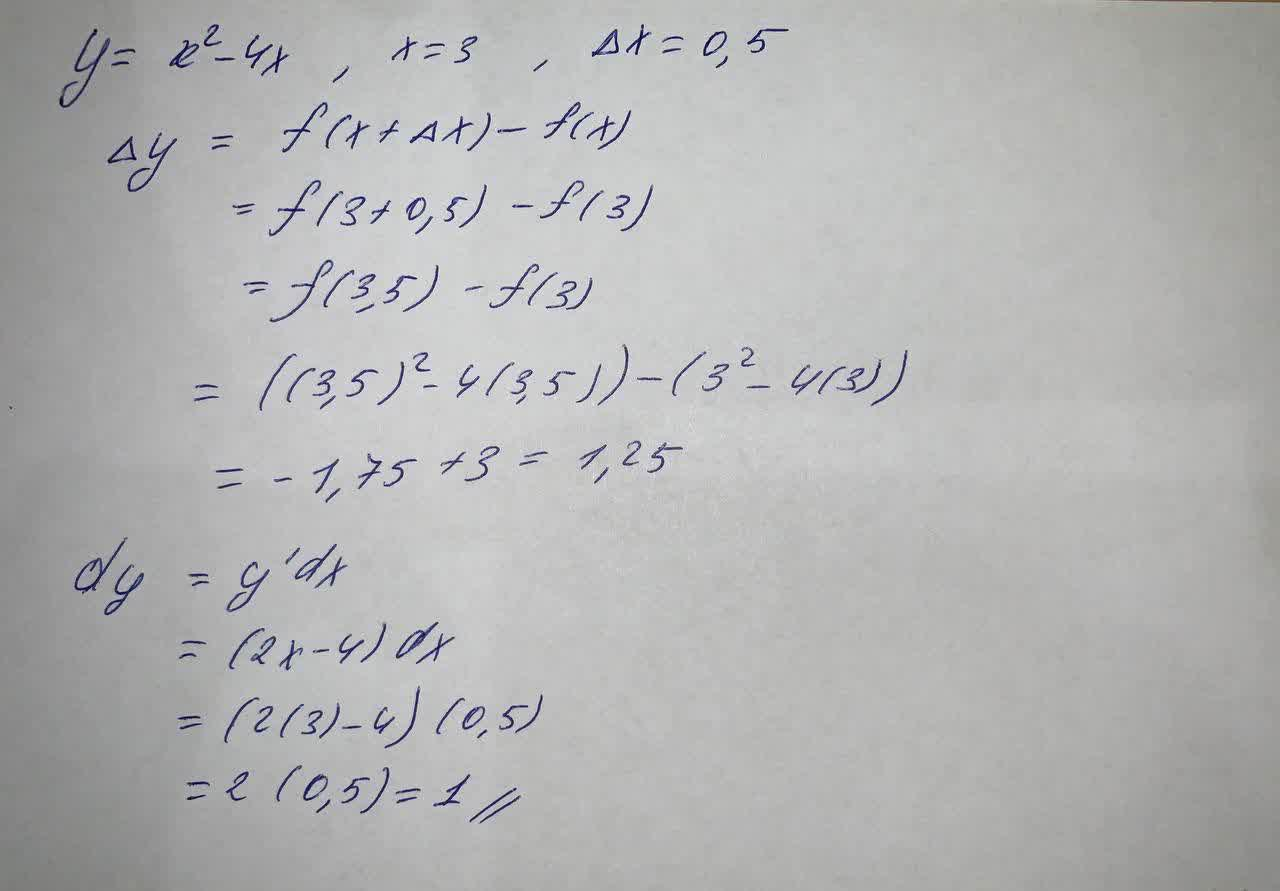# Compute \triangle y and dy for the given values of x and dx=\triangle x PSmidtlinjeg 2021-09-20 Answered
Compute $$\displaystyle\triangle{y}$$ and dy for the given values of x and $$\displaystyle{\left.{d}{x}\right.}=\triangle{x}$$
$$\displaystyle{y}={x}^{{2}}-{4}{x},{x}={3},\triangle{x}={0},{5}$$
$$\displaystyle\triangle{y}=???$$
dy=?

• Questions are typically answered in as fast as 30 minutes

### Plainmath recommends

• Get a detailed answer even on the hardest topics.
• Ask an expert for a step-by-step guidance to learn to do it yourself.firmablogF
To compute: